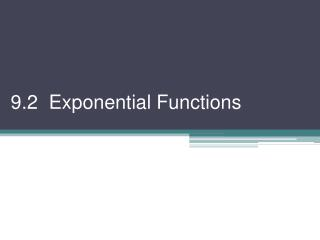DownloadDownload Presentation9.2 Exponential Functions

# 9.2 Exponential Functions

Download Presentation## 9.2 Exponential Functions

- - - - - - - - - - - - - - - - - - - - - - - - - - - E N D - - - - - - - - - - - - - - - - - - - - - - - - - - -
##### Presentation Transcript

1. 9.2 Exponential Functions

2. A function that can be expressed in the form f (x) = bx, b > 0 and b ≠ 1 is called an exponential function Domain =  Range = y > 0 (positive Real #s) To get an idea of their basic shape, let’s graph 2 using a table of values: Ex 1) a) h(x) = 2x b) g(x) = 5x x y x y –2 2–2= –2 ¼ 1/25 26 –1 2–1= ½ –1 1/5 0 20 = 1 0 1 2 1 21 = 5 1 22 = 2 4 2 25 23 = 3 8 3 125 R: y > 0, x-int: none D: ,  same one-to-one y-int: (0, 1), increasing,

3. Remember that if you have f (x), then f (–x) is the reflection of f (x) over the y-axis. Ex 2) Graph We can write this as f (x) = (2–1)x = 2–x It is the graph in Ex 1a) flipped over the y-axis *Note: Any function of the form f (x) = cx is one-to-one *We can reflect, dilate, & translate exponential functions – just like all our other functions. The natural exponential function is f (x) = ex e is defined as the number that approaches as n approaches infinity e ≈ 2.718281828….

4. Ex 3) The graph of f (x) = ex is “Two Truths & a Lie” Thinking about transformations, which of these is false? Why? a) h(x) = e–x b) j(x) = –ex c) k(x) = –e–x How about this set – which is false? Why? a) p(x) = ex + 2 b) q(x) = ex+2 c) r(x) = ex–2

5. Exponential Equations *Hints: write both expressions with the same base or factor Ex 4) Solve a) 43x+1 = 32 b) x2e3x = 4e3x x2e3x – 4e3x = 0 e3x(x2 – 4) = 0 e3x = 0 x2 – 4 = 0 (can’t be 0) x2 = 4 x = ±2 change to base 2: 22(3x+1) = 25 2(3x+1)= 5 6x + 2 = 5 6x = 3 x = ½ exponents:

6. Populations: Many populations are modeled using exponential equations. In general, P = P0(1 + r)t Ex 5) The US Census says the population in 1960 was 179,000,000 and was 203,000,000 in 1970. Determine a model & estimate the population in 1975. To find r we need the increase: 1970 pop. – 1960 pop. = 203,000,000 – 179,000,000 = 24,000,000 This is per 10 years, so per year: so P = 179,000,000(1 + 0.013)t In 1975, P = 179,000,000(1 + 0.013)15 ≈ 217,000,000

7. Homework #902 Pg 450 #1, 3, 5, 7, 11, 15, 17–24, 30–34, 38, 41, 46–48